• 定义：由 m × n 个数aij排成的m行n列的数表称为m行n列的矩阵，简称 m × n矩阵；在编程语言中可以当做二维数组来处理； 加减法：同型矩阵之间 ...转置：把矩阵A的行换成同序数的列所得到的新矩阵称为A的转置矩阵
定义：由 m × n 个数aij排成的m行n列的数表称为m行n列的矩阵，简称             m × n矩阵；在编程语言中可以当做二维数组来处理； 加减法：同型矩阵之间 乘法：两个矩阵的乘法仅当第一个矩阵A的列数和另一个矩阵B的行数相等时才能定义。如A是m×n矩阵和B是n×p矩阵，它们的乘积C是一个m×p矩阵 ； 转置：把矩阵A的行换成同序数的列所得到的新矩阵称为A的转置矩阵；
详细的运算及原理可以百度一下；
如下是完整的Java代码实现：

package algorithm;

public class Matrix {
public final int TYPE_ADD = 1;
public final int TYPE_MUL = 2;

/**
* 矩阵类，实现n阶矩阵的加、乘、转置运算
*
* @param args
*/
public static void main(String[] args) {
// TODO Auto-generated method stub
int[][] a = { { 1, 2 }, { 2, 3 } };
int[][] b = { { 4, 5 }, { 6, 7 } };
int[][] aa = new int[][]{{1,0,2},{-1,3,1}};
int[][] bb = new int[][]{{3,1}, {2,1},{1,0}};
Matrix m = new Matrix();

System.out.println("两矩阵相加：");
int[][] r = m.matrix_add(a, b);
for (int i = 0; i < r.length; i++) {
for (int j = 0; j < r[i].length; j++) {
System.out.print(r[i][j] + "\t");
}
System.out.println();
}

System.out.println("两矩阵相乘：");
r = m.matrix_mul(aa, bb);
for (int i = 0; i < r.length; i++) {
for (int j = 0; j < r[i].length; j++) {
System.out.print(r[i][j] + "\t");
}
System.out.println();
}

System.out.println("矩阵转置：");
r = m.matrix_tran(a);
for (int i = 0; i < r.length; i++) {
for (int j = 0; j < r[i].length; j++) {
System.out.print(r[i][j] + "\t");
}
System.out.println();
}

}

// 矩阵相加
public int[][] matrix_add(int[][] a, int[][] b) {
int[][] c = new int[a.length][a.length];
if (inputLegal(a, b, TYPE_ADD)) {// 只有同型矩阵才能相加
for (int i = 0; i < a.length; i++) {// i控制行
for (int j = 0; j < a[i].length; j++) {// j控制列
c[i][j] = a[i][j] + b[i][j];
}
}
}
return c;
}

// 矩阵相乘
public int[][] matrix_mul(int[][] a, int[][] b) {
int[][] d = new int[a.length][b.length];
if (inputLegal(a, b, TYPE_MUL)) {// 两个矩阵的乘法仅当第一个矩阵A的行数和另一个矩阵B的列数相等时才能定义
for (int i = 0; i < a.length; i++) {// 最终结果矩阵为A的行
for (int j = 0; j < b[i].length; j++) {// 最终结果矩阵为B的列
/*
* 罗列系数之后会发现如下规律，a的行标记随i，b的列标记随j，而a的列标记和b的行标记一样，且从0到n，
* 其中n为a的列数，故还需要一个变量k来循环控制这个从0到n的标记
*/
int num = 0;
for (int k = 0; k < a[i].length; k++) {
num += a[i][k] * b[k][j];
}
d[i][j] = num;
}
}
}
return d;
}

//矩阵转置
public int[][] matrix_tran (int[][] a) {
//矩阵A的行换成同序数的列所得到的新矩阵称为A的转置矩阵
int[][] e = new int[a.length][a.length];
for (int i = 0; i < a.length; i++) {
for (int j = 0; j< a.length; j++) {
e[j][i] = a[i][j];
}
}
return e;
}

// 矩阵类型校验
public boolean inputLegal(int[][] a, int[][] b, int type) {
boolean flag = true;
if (type == TYPE_ADD) {
if (a.length != b.length || a.length != b.length) {
flag = false;// 判断是否为同型矩阵
}
}
if (type == TYPE_MUL) {
if (a.length != b.length) {
flag = false;// 判断一个矩阵的行与另一个矩阵的列是否相等
}
}
return flag;
}
}


期待你的更高效代码！
展开全文算法
• 1、矩阵乘 1） 矩阵乘A*B 是以数学运算中矩阵相乘方式...如A是m×n矩阵，B是n×p矩阵，它们乘积AB是一个m×p矩阵。 例如： Mat A=Mat::ones(2,3,CV_32FC1); Mat B=Mat::ones(3,2,CV_32FC1); ... AB=A*B; 2） ...
1、矩阵加
1) 使用重载的 “+” 运算符
矩阵的加法是指两个矩阵对于位置的数值相加，使用OpenCv重载的 “+” 运算符,假设两个矩阵都为uchar类型,例如：
Mat src1 = (Mat_<uchar>(2,3) << 23, 123, 90, 100, 250, 0);
Mat src2 = (Mat_<uchar>(2,3) << 125, 150, 60, 100, 10, 40);
Mat dst = src1 + src2;

123+150应该等于273, 因为两个矩阵的类型都是uchar, 所以"+“运算计算出来的和也是uchar类型, 但是uchar类型范围的最大值是255, 所以273限制为了255。
利用”+“运算符计算计算Mat的和需注意:两个Mat的数据类型必须是一样的, 否则会报错。
一个数值与一个Mat对象相加, 也可以使用”+"运算符, 但是无论这个数值是什么数据类型, 返回的Mat的数据类型都与输入的Mat相同，且结果是矩阵中的每个元素都与这个数值相加:
例如：
float value = 100.0;
Mat dst1 = src1 + value;

2) 使用add函数
void add
(
InputArray src1,
InputArray src2,
OutputArray dst,
InputArray mask = noArray(),
int dtype = -1
);

例如：
Mat src1 = (Mat_<uchar>(2,3) << 23, 123, 90, 100, 250, 0);
Mat src2 = (Mat_<uchar>(2,3) << 125, 150, 60, 100, 10, 40);
Mat dst;
add(src1, src2, dst, Mat(), CV_64FC1);

使用add函数时, 输入矩阵的数据类型可以不同, 二输出矩阵的数据类型可以根据情况自行指定, 只有当src1和src2的数据类型相同时, 才能令dtype = -1, 否则仍然会报错。
3) 两个向量之间也可以做加法运算
Vec3f v1 = Vec3f(1, 2, 3);
Vec3f v2 = Vec3f(10, 1, 12);
Vec3f v = v1 + v2;

4）线性相加scaleAdd
C++: void scaleAdd
(
InputArray src1,     // 第一个输入矩阵
double alpha,        // 第一个输入矩阵的比例因子
InputArray src2,     // 与src1大小和类型相同的第二个输入矩阵。
OutputArray dst
)

它计算一个缩放数组和另一个数组的和:
dst(I) = alpha * src(I) + src2(I)
例如：
scaleAdd(imageA,k,imageB,resultC);

该函数也可以用矩阵表达式模拟，例如：
Mat A(3, 3, CV_64F);
...
A.row(0) = A.row(1)*2 + A.row(2);

5）两个数组的加权和addWeighted
C++: void addWeighted
(
InputArray src1,      // 第一个图像矩阵
double alpha,         // 第一个数组元素的权重。
InputArray src2,      // 与src1大小和通道相同的第二个输入图像矩阵。
double beta,          // 第二个数组元素的权值。
double gamma,         // 与输入数组具有相同大小和通道数的输出数组。
OutputArray dst,      // 每个和加上一个标量。
int dtype=-1          // 可选输出阵列深度;当两个输入数组具有相同的
// 深度时，可以将dtype设置为-1，这将等效于src1.depth()。
)

该函数可替换为矩阵表达式:
dst = src1 * alpha + src2 * beta + gamma;
例如：
	Mat img1=imread("./a1.jpg");
Mat img2=imread("./a2.jpg");
Mat dst;
addWeighted(img1,0.5,img2,0.3,0,dst);

2、矩阵减
1）使用重载“-”运算符
我们可以使用和**"-"**符号进行 矩阵减运算。
例如：
Mat a= Mat::eye(Size(3,2), CV_32F);
Mat b= Mat::ones(Size(3,2), CV_32F);
Mat d= a-b;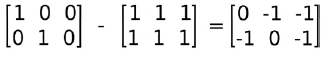注：如果图像是uchar类型的，144-240不会是 - 96，而是uchar能表示的最小值0

2）减法函数subtract
计算两个数组或数组与标量之间的每个元素的差。
C++: void subtract
(
InputArray src1,           // 第一个输入数组或标量。
InputArray src2,           // 第二个输入数组或标量。
OutputArray dst,           // 与输入阵列相同大小、相同通道数的输出阵列。
InputArray mask=noArray(), // 可选操作面具;这是一个8位的单通道数组，
// 指定要更改的输出数组的元素。
int dtype=-1               // 输出数组的可选深度
)

例如：
imshow("img1",img1);
imshow("img2",img2);
subtract(img1,img2,dst);    // saturate(img1 - img2)
//注意：要求被处理图片尺寸一致


注：图像元素类型是uchar，如果相减小于0，结果值会变成0，如果大于255，变成255。

3) 元素的绝对差absdiff
计算两个数组或数组与标量之间的每个元素的绝对差。
C++: void absdiff
(
InputArray src1,    // 第一个输入数组或标量。
InputArray src2,    // 第二个输入数组或标量。
OutputArray dst     // 与输入数组具有相同大小和类型的输出数组。
)

dst = saturate(|src1 - src2|)
例如：
imshow("img1",src1);
imshow("img1",src2);
Mat dst;
absdiff(src1, src2, dst);//若dst<0,则dst=|dst|>=0

3、矩阵乘
1） 矩阵乘A*B
是以数学运算中矩阵相乘的方式实现的，即Mat矩阵A和B被当做纯粹的矩阵做乘法运算，这就要求A的列数等于B的行数时，才能定义两个矩阵相乘。如A是m×n矩阵，B是n×p矩阵，它们的乘积AB是一个m×p矩阵。
例如：
Mat A=Mat::ones(2,3,CV_32FC1);
Mat B=Mat::ones(3,2,CV_32FC1);
...
AB=A*B;

2） 点乘A.dot(B)（与MATLAB的概念有点区别）
参与点乘的两个Mat矩阵的数据类型（type）只能是 CV_32F、 CV_64FC1、 CV_32FC2、 CV_64FC2 这4种类型中的一种。若选用其他类型，比如CV_8UC1，编译器会报错。
说明： 对于向量a和向量b：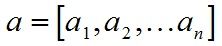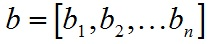a和b的点积公式为：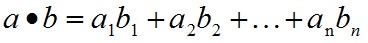A.dot(B)操作相当于数学向量运算中的点乘，也叫向量的内积、数量积。 对两个向量执行点乘运算，就是对这两个向量对应位一一相乘之后求和的操作，点乘的结果是一个标量。 Mat矩阵的dot方法扩展了一维向量的点乘操作，把整个Mat矩阵扩展成一个行（列）向量，之后执行向量的点乘运算，仍然要求参与dot运算的两个Mat矩阵的行列数完全一致。
dot方法声明中显示返回值是double，所以A.dot(B)结果是一个double类型数据，不是Mat矩阵，不能把A.dot(B)结果赋值给Mat矩阵！
例如：
Mat A=Mat::ones(2,3,CV_8UC1);
Mat B=Mat::ones(2,3,CV_8UC1);
double AB;
...
AB = A.dot(B);

dot操作不对参与运算的矩阵A、B的数据类型做要求，CV_8UC1、CV_32FC1等，可以是任何Opencv定义的类型，如在2中使用的就是CV_8UC1。
若参与dot运算的两个Mat矩阵是多通道的，则计算结果是所有通道单独计算各自.dot之后，再累计的和，结果仍是一个double类型数据。
3）  计算两个Mat矩阵对应位的乘积A.mul(B)
要求参与运算的矩阵A的行列和B的行列数一致。计算结果是跟A或B行列数一致的一个Mat矩阵。
mul说明：

1、mul操作不对参与运算的两个矩阵A、B有数据类型上的要求，但要求A，B类型一致，不然报错；
2、Mat AB=A.mul(B)，若声明AB时没有定义AB的数据类型，则默认AB的数据类型跟A和B保存一致；
3、若AB精度不够，可能产生溢出，溢出的值被置为当前精度下的最大值；

例如：
Mat A=Mat::ones(2,3,CV_8UC1);
Mat B=Mat::ones(2,3,CV_8UC1);
...
Mat AB=A.mul(B);

4） 矩阵与标量相乘
使用“*”示矩阵与标量相乘；
例如：
Mat m1 = Mat::eye(2,3,CV_32FC1);
...
Mat m2 = m1*2;

4、矩阵除
1）使用重载的 “/” 运算符
A/B; alpha/A; A/alpha都是点除
例如：
Mat src1 = (Mat_<int>(2, 3) << 4, 7, 1, 5, 20, 24);  //2行3列的矩阵
Mat src2 = (Mat_<int>(2, 3) << 2, 7, 5, 5, 4, 48);  //2行3列的矩阵
Mat dst1, dst2, dst3;
dst1 = src1 / 4;
dst2 = 20 / src1;
dst3 = src1 / src2;
cout << "A/alpha:\n" << dst1 << endl << endl;
cout << "alpha/A:\n" << dst2 << endl << endl;
cout << "A/B:\n" << dst3 << endl;

输出结果：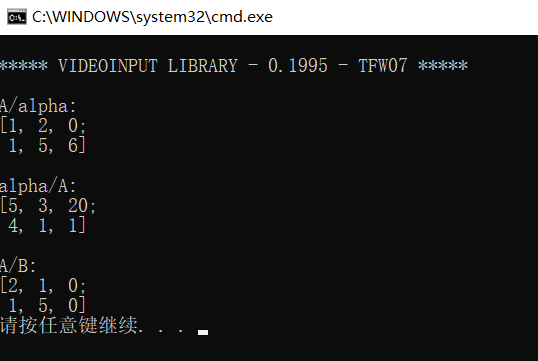2）矩阵除函数 divide
对一个数组执行两个数组或一个标量的每个元素的除法。
C++: void divide
(
InputArray src1,     // 第一个输入矩阵
InputArray src2,     // 与src1大小和类型相同的第二个输入矩阵。
OutputArray dst,     // 与src2大小和类型相同的输出矩阵。
double scale=1,      // 标量的因子
int dtype=-1
)

函数将一个数组除以另一个数组:
dst = saturate(src1*scale/src2)
如果没有src1时：
dst = saturate(scale/src2);
例如：
Mat img0 = cv::imread("img_0.jpg", -1);
Mat img1 = cv::imread("img_1.jpg", -1);
Mat img2;
divide(img0, img1, img2, 50, -1);

5、矩阵的转置
由Mat类t()函数实现矩阵的转置
例如：
Mat m1 = Mat::eye(4,6,CV_32FC1);
...
Mat m1t = m1.t();

6、矩阵的逆
其中inv(A)表示矩阵A的逆矩阵
例如：
Mat m1 = Mat::eye(5,5,CV_32FC1);
...
Mat m1inv = m1.inv();

7、矩阵中非零元素个数
使用 countNonZero() 函数实现物体的像素或面积常需要用到计算矩阵中的非零元素个数
例如：
Mat m1 = Mat::eye(6,6,CV_32FC1);
...
int m1num = countNonZero(m1);

8、矩阵中均值和标准差
OpenCV提供了矩阵均值和标准差计算功能，使用 meanStdDev(src,mean,stddev) 函数实现
例如：
Mat m1 = Mat::eye(5,5,CV_32FC1);
Mat mean,stddev;
...
meanStdDev(m1,mean,stddev);
.
.
.
Mat m3(Size(5,5),CV_8UC3,Scalar(255,200,100));
Mat mean3,stddev3;
...
meanStdDev(m3,mean3,stddev3);


注：当src为多通道或多维矩阵时，则函数分别计算不同通道的均值与标准差，因此返回的mean和stddev为对应维度的向量；


展开全文• C#矩阵运算（加减、转置、求逆）   class Matrix ... /// 矩阵的转置 /// &lt;/summary&gt; /// &lt;param name= "iMatrix "&gt; &lt;/param&gt; p...
C#矩阵运算（加减乘、转置、求逆）

class Matrix
{

///   <summary>
///   矩阵的转置
///   </summary>
///   <param   name= "iMatrix "> </param>
public static double[,] Transpose(double[,] iMatrix)
{
int row = iMatrix.GetLength(0);
int column = iMatrix.GetLength(1);
//double[,] iMatrix = new double[column, row];
double[,] TempMatrix = new double[row, column];
double[,] iMatrixT = new double[column, row];
for (int i = 0; i < row; i++)
{
for (int j = 0; j < column; j++)
{
TempMatrix[i, j] = iMatrix[i, j];
}
}
for (int i = 0; i < column; i++)
{
for (int j = 0; j < row; j++)
{
iMatrixT[i, j] = TempMatrix[j, i];
}
}
return iMatrixT;

}

///   <summary>
///   矩阵的逆矩阵
///   </summary>
///   <param   name= "iMatrix "> </param>
public static double[,] Athwart(double[,] iMatrix)
{
int i = 0;
int row = iMatrix.GetLength(0);
double[,] MatrixZwei = new double[row, row * 2];
double[,] iMatrixInv = new double[row, row];
for (i = 0; i < row; i++)
{
for (int j = 0; j < row; j++)
{
MatrixZwei[i, j] = iMatrix[i, j];
}
}
for (i = 0; i < row; i++)
{
for (int j = row; j < row * 2; j++)
{
MatrixZwei[i, j] = 0;
if (i + row == j)
MatrixZwei[i, j] = 1;
}
}

for (i = 0; i < row; i++)
{
if (MatrixZwei[i, i] != 0)
{
double intTemp = MatrixZwei[i, i];
for (int j = 0; j < row * 2; j++)
{
MatrixZwei[i, j] = MatrixZwei[i, j] / intTemp;
}
}
for (int j = 0; j < row; j++)
{
if (j == i)
continue;
double intTemp = MatrixZwei[j, i];
for (int k = 0; k < row * 2; k++)
{
MatrixZwei[j, k] = MatrixZwei[j, k] - MatrixZwei[i, k] * intTemp;
}
}
}

for (i = 0; i < row; i++)
{
for (int j = 0; j < row; j++)
{
iMatrixInv[i, j] = MatrixZwei[i, j + row];
}
}
return iMatrixInv;
}

///   <summary>
///   矩阵加法
///   </summary>
///   <param   name= "MatrixEin "> </param>
///   <param   name= "MatrixZwei "> </param>
public static double[,] AddMatrix(double[,] MatrixEin, double[,] MatrixZwei)
{
double[,] MatrixResult = new double[MatrixEin.GetLength(0), MatrixZwei.GetLength(1)];
for (int i = 0; i < MatrixEin.GetLength(0); i++)
for (int j = 0; j < MatrixZwei.GetLength(1); j++)
MatrixResult[i, j] = MatrixEin[i, j] + MatrixZwei[i, j];
return MatrixResult;
}

///   <summary>
///   矩阵减法
///   </summary>
///   <param   name= "MatrixEin "> </param>
///   <param   name= "MatrixZwei "> </param>
public static double[,] SubMatrix(double[,] MatrixEin, double[,] MatrixZwei)
{
double[,] MatrixResult = new double[MatrixEin.GetLength(0), MatrixZwei.GetLength(1)];
for (int i = 0; i < MatrixEin.GetLength(0); i++)
for (int j = 0; j < MatrixZwei.GetLength(1); j++)
MatrixResult[i, j] = MatrixEin[i, j] - MatrixZwei[i, j];
return MatrixResult;
}

///   <summary>
///   矩阵乘法
///   </summary>
///   <param   name= "MatrixEin "> </param>
///   <param   name= "MatrixZwei "> </param>
public static double[,] MultiplyMatrix(double[,] MatrixEin, double[,] MatrixZwei)
{
double[,] MatrixResult = new double[MatrixEin.GetLength(0), MatrixZwei.GetLength(1)];
for (int i = 0; i < MatrixEin.GetLength(0); i++)
{
for (int j = 0; j < MatrixZwei.GetLength(1); j++)
{
for (int k = 0; k < MatrixEin.GetLength(1); k++)
{
MatrixResult[i, j] += MatrixEin[i, k] * MatrixZwei[k, j];
}
}
}
return MatrixResult;
}

///   <summary>
///   矩阵对应行列式的值
///   </summary>
///   <param   name= "MatrixEin "> </param>
///   <returns> </returns>
public static double ResultDeterminant(double[,] MatrixEin)
{
return MatrixEin[0, 0] * MatrixEin[1, 1] * MatrixEin[2, 2] + MatrixEin[0, 1] * MatrixEin[1, 2] * MatrixEin[2, 0] + MatrixEin[0, 2] * MatrixEin[1, 0] * MatrixEin[2, 1]
- MatrixEin[0, 2] * MatrixEin[1, 1] * MatrixEin[2, 0] - MatrixEin[0, 1] * MatrixEin[1, 0] * MatrixEin[2, 2] - MatrixEin[0, 0] * MatrixEin[1, 2] * MatrixEin[2, 1];

}

}


展开全文• 两个矩阵A和B，存在满秩矩阵P，P的转置乘AP等于B，二者合同。 特殊考虑情况 对称矩阵的不同的特征值对应的特征向量必定正交 存在正交矩阵Q-1AQ=B，使A和B相似 Q-1等价于Q的转置 所以A和B合同。 合同、等价和...
相似的两个条件

特征值相同
均可对角化

特征值相同而且均可对角化 的话，不就都可以对角化为一个对角矩阵（对角元为特征值）  A~B  C~B  则A~C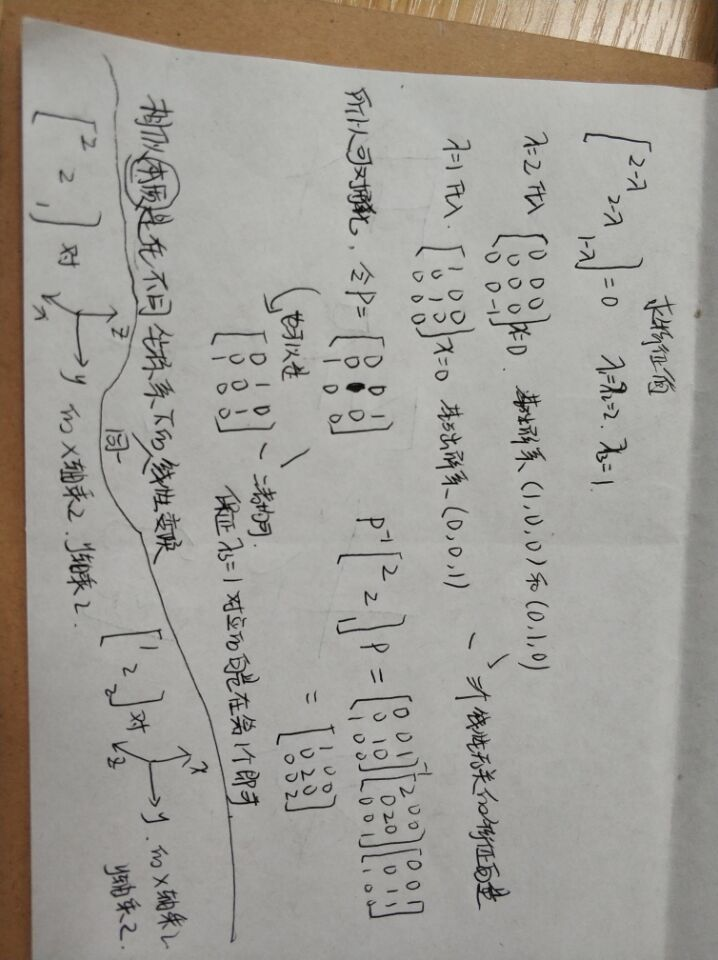合同的条件
两个矩阵A和B，存在满秩矩阵P，P的转置乘A乘P等于B，二者合同。
特殊考虑情况
对称矩阵的不同的特征值对应的特征向量必定正交
存在正交矩阵Q-1AQ=B，使A和B相似
Q-1等价于Q的转置
所以A和B合同。
合同、等价和相似的区别
1.矩阵相似的例子中，P-1AP=B；针对方阵而言；秩相等为必要条件；本质是二者有相等的不变因子；可看作是同一线性变换在不同基下的矩阵；矩阵相似必等价，但等价不一定相似。

矩阵合同的例子中，CTAC=B；针对方阵而言；秩相等为必要条件；本质是秩相等且正惯性指数相等，即标准型相同；可通过二次型的非退化的线性替换来理解；矩阵合同必等价，但等价不一定合同。

正交矩阵
1、方阵A正交的充要条件是A的行（列）向量组是单位正交向量组；
2、方阵A正交的充要条件是A的n个行（列）向量是n维向量空间的一组标准正交基；
3、A是正交矩阵的充要条件是：A的行向量组两两正交且都是单位向量；
4、A的列向量组也是正交单位向量组；
5、正交方阵是欧氏空间中标准正交基到标准正交基的过渡矩阵。
特征向量正交化
因为特征向量的正交化是局限在同一特征值的特征向量，特征向量是对应齐次线性方程组的解，所以特征向量的非零线性组合仍是特征向量。正交化所得向量与原向量等价，所以仍是特征向量，由此可知单位化后也是特征向量。
当同一个特征值a是两个基础解系的线性组合时，说明对于这个线性组合的任意向量都是收缩a倍


展开全文线性代数
• （a,b）,(c.d)视为两个行向量三节行列式求解：通用定义：代数...矩阵转置不影响行列式值。注意是单行：来自为知笔记(Wiz) 转载于:https://www.cnblogs.com/iathena/p/28093e8baa13ed0ef880b3418a515406.html...
• 0.菜鸟预知识 0.1矩阵 定义： ...如A是m×n矩阵和B是n×p矩阵，它们乘积C是一个m×p矩阵 ，它一个元素：例： 0.3行列式 一个n×n正方矩阵A行列式记为det(A)或者|A| ,一个2×2矩阵的行列式可
• 本节引入向量空间和子空间~ 置换与转置 置换： Permutations 记为P，是通过对单位矩阵进行行变换得到的。 前面用消元法解线性方程组时：...矩阵A的转置记为,将矩阵的行变为列，看起来像是沿着对角线进行翻转。 ...线性代数
• 任务：模拟矩阵运算，支持矩阵转置，修改矩阵大小，矩阵与数字加、减、运算，以及矩阵矩阵的加、减、运算。 1 class simNumpyArray(object): 2 3 def __init__(self,p): 4 '''可以接收列表、...
• 转：https://www.jianshu.com/p/e969a50bb0a5?utm_campaign=maleskine&utm_content=note&utm_medium=seo_notes&utm_source=recommendation 考虑矩阵的特征值。 若所有特征值均不小于零，则...A的转置乘...
• 头文件，包含矩阵 【创建、叉乘、读取、修改、加减、转置 】操作 //文件名：mat.h #ifndef _H_MAT #define _H_MAT #define RIGHT true//右 #define LEFT false//左 typedef struct mat{ int colSize;//行大小...线性代数
• 文章目录前置技能行列式定义性质拉普拉斯展开线性性可性可加性不重性可倍加性转置不变性可交换性行可交换性列可交换性优化行列式计算矩阵树定理前置定义一些引理转置引理连通性引理引理 1引理 2引理 3Binet - ...线性代数
• 设 $B=AA^T$ ，其中 $A^T$ 为 $A$ 的转置矩阵，那么对于 $B_{i,j}$ 的值，它其实就是向量 $i$ 和向量 $j$ 的内积 注意到 $K$ 只有 $2$ 或 $3$，先考虑 $K=2$ 时的情况 此时就是问矩阵 $B$ 在模 \$...
• 顶点坐标向量vertexPosition左MVP矩阵（注意顺序，若右，根据矩阵乘法性质则为各自转置后倒序相乘），gl_Position=P(Projection)V(View)M(Model)vertexPosition，其中，M(Model)=T(Translate)R(Rotat...OpenGL
• 协方差矩阵 cov(X) 相关系数矩阵 corrcoef(X) 设原矩阵 X（n,p），标准化矩阵 Xstd（n,p...2.标准化矩阵转置乘本身 == 标准化矩阵的协方差矩阵的（n-1）倍 即：Xstd'*Xstd ==（n-1）*cov（Xstd）==（n-1）*corrco...
• 1） 求稀疏矩阵加，转置矩阵。 2） 求广义表深度。 实验6：树和二叉树 1） 求赫夫曼编码。（w存放n个字符权值（均>0），构造赫夫曼树HT，并求出n个字符赫夫曼编码HC） 实验7：图 1）实现教科书中图...数据结构
• 写一函数，将一个33整型矩阵转置 开始自己写一直转置不成功，因为在转置第二层循环那里写j<3这样前面转置的又转回去了，把那个条件改一下就好了。 #include<stdio.h> //自己写 //#if 0 int main...程序设计 c语言 指针
• 特征降维：特征选择和特征...对于一个矩阵X，用X乘以X的转置，并上系数1/m，矩阵对角线上的两个元素分别是两个字段的方差，而其他元素是a和b的协方差，两者 被统一到一个矩阵当中。 优化目标变成了一个寻找一个矩阵p
• 向量或者矩阵的转置 ⊙\odot⊙ 按元素相乘 ∥⋅∥p\|\cdot\|_p∥⋅∥p​ LpL_pLp​范数 ∥⋅∥\|\cdot\|∥⋅∥ L2L_2L2​范数 ∑\sum∑ 连加 ∏\prod∏ 连 f(⋅)f(\cdot)f(⋅) 函数C语言神经网络框架 符号对照表
• (g) 稀疏矩阵的转置![图片说明](https://img-ask.csdn.net/upload/201812/24/1545641300_687295.png) 链表上的操作。 #include"pch.h" #include #include #include #include #define OK 1 #define ERROR ...c++
• 1） 求稀疏矩阵加，转置矩阵。 2） 求广义表深度。 实验6：树和二叉树 1） 求赫夫曼编码。（w存放n个字符权值（均>0），构造赫夫曼树HT，并求出n个字符赫夫曼编码HC） 实验7：图 1）实现教科书中图...数据结构
• 第四章向量和矩阵4·1Rn中的向量4·2矩阵矩阵加法和数4·3矩阵乘法4·4矩阵的转置4·5方阵4·6特殊类型的方阵4·7行列式 第五章图论5·1图和多重图5·2一个顶点的次数5·3通路、连通性5·4子图、连通分图、割点...